# Optimizing Memory Architecture - 2022.1 English

## Vitis Unified Software Platform Documentation: Application Acceleration Development (UG1393)

Document ID
UG1393
Release Date
2022-05-25
Version
2022.1 English

Memory architecture is a key aspect of implementation. Due to the limited access bandwidth, it can heavily impact the overall performance, as shown in the following example:

``````
void run (ap_uint<16> in,
ap_uint<16> out
) {
...
ap_uint<16> inMem;
ap_uint<16> outMem;

... Preprocess input to local memory

for( int j=0; j<256; j++) {
#pragma HLS PIPELINE OFF
ap_uint<16> sum = 0;
for( int i = 0; i<4; i++) {

sum += inMem[j][i];
}
outMem[j] = sum;
}

... Postprocess write local memory to output
}``````

This code adds the four values associated with the inner dimension of the two dimensional input array. If implemented without any additional modifications, it results in the following estimates:

Figure 1. Performance Estimates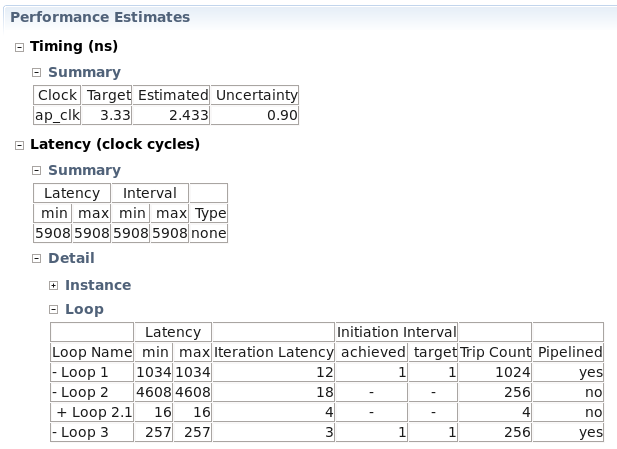The overall latency of 4608 (Loop 2) is due to 256 iterations of 18 cycles (16 cycles spent in the inner loop, plus the reset of sum, plus the output being written). This is observed in the Schedule Viewer in the HLS Project. The estimates become considerably better when unrolling the inner loop.

Figure 2. Performance Estimates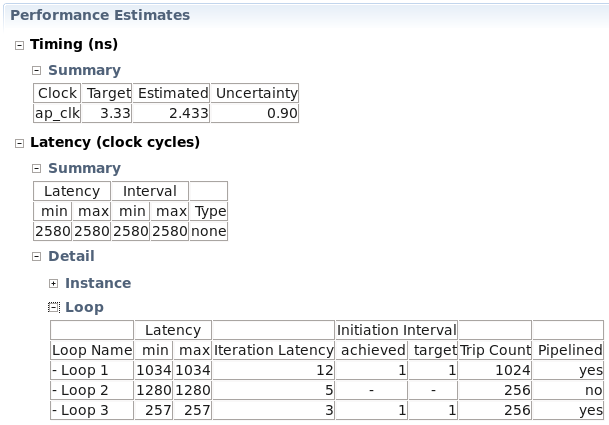However, this improvement is largely because of the process using both ports of a dual port memory. This can be seen from the Schedule Viewer in the HLS Project:

Figure 3. Schedule Viewer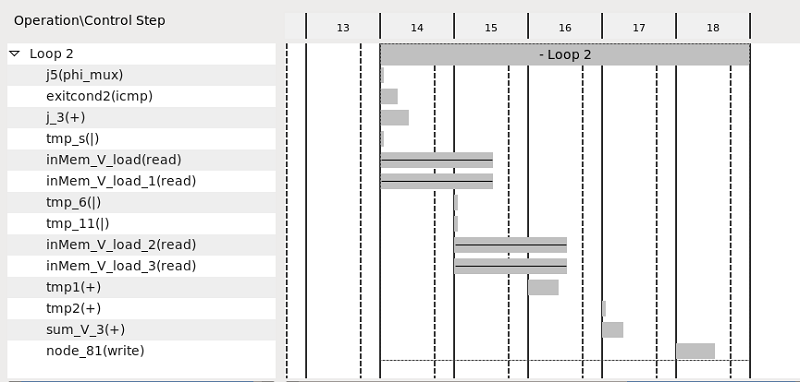Two read operations are performed per cycle to access all the values from the memory to calculate the sum. This is often an undesired result as this completely blocks the access to the memory. To further improve the results, the memory can be split into four smaller memories along the second dimension:

``#pragma HLS ARRAY_PARTITION variable=inMem complete dim=2``

This results in four array reads, all executed on different memories using a single port:

Figure 4. Executed Four Arrays Results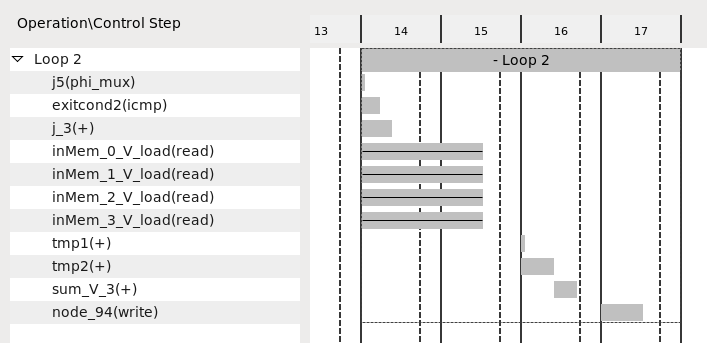Using a total of 256 * 4 cycles = 1024 cycles for loop 2.

Figure 5. Performance Estimates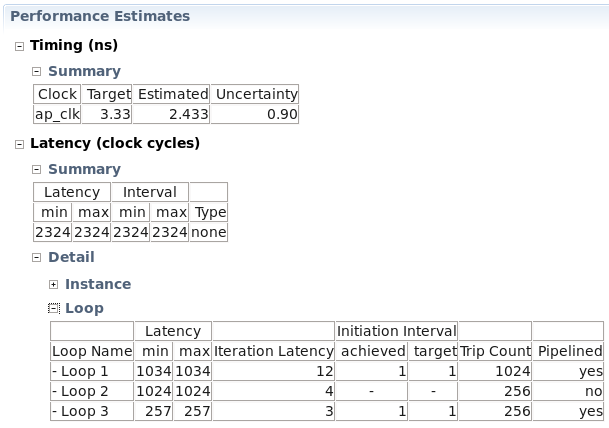Alternatively, the memory can be reshaped into to a single memory with four words in parallel. This is performed through the pragma:

``#pragma HLS array_reshape variable=inMem complete dim=2``

This results in the same latency as when the array partitioning, but with a single memory using a single port:

Figure 6. Latency Result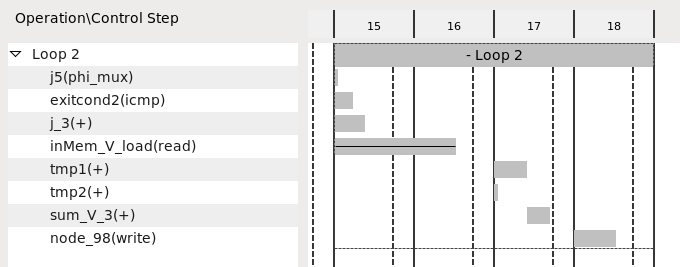Although, either solution creates comparable results with respect to overall latency and utilization, reshaping the array results in cleaner interfaces and less routing congestion making this the preferred solution.

Note: This completes array optimization, in a real design the latency could be further improved by employing loop parallelism (see Loop Parallelism).
``````void run (ap_uint<16> in,
ap_uint<16> out
) {
...

ap_uint<16> inMem;
ap_uint<16> outMem;
#pragma HLS array_reshape variable=inMem complete dim=2

... Preprocess input to local memory

for( int j=0; j<256; j++) {
#pragma HLS PIPELINE OFF
ap_uint<16> sum = 0;
for( int i = 0; i<4; i++) {
#pragma HLS UNROLL
sum += inMem[j][i];
}
outMem[j] = sum;
}

... Postprocess write local memory to output

}``````